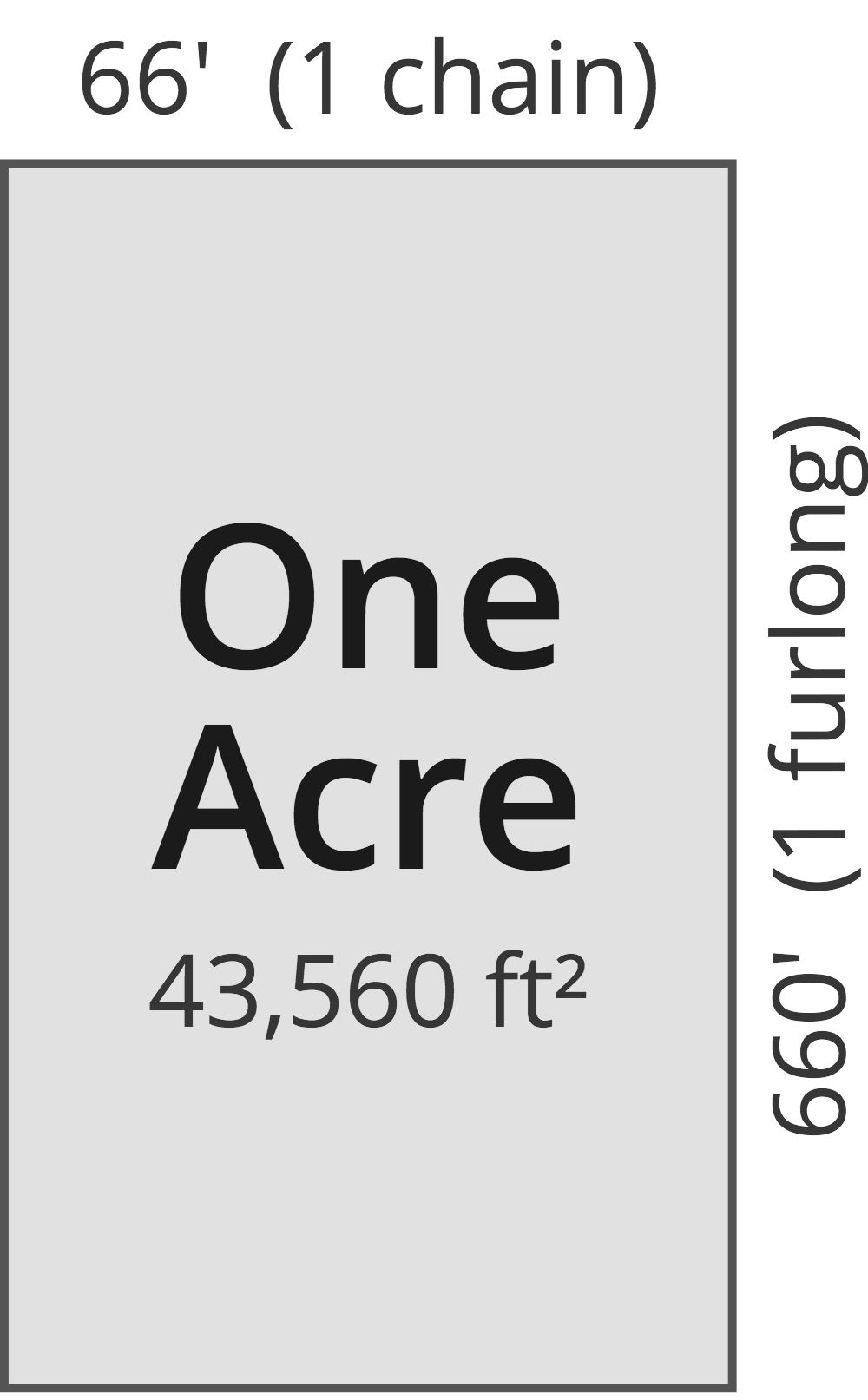# Acres to Square Meters Converter

Enter the area in acres below to get the value converted to square meters.

(find acres)
Results in Square Meters:1 ac = 4,046.856422 sq m

Do you want to convert square meters to acres?

## How to Convert Acres to Square Meters

To convert a measurement in acres to a measurement in square meters, multiply the area by the following conversion ratio: 4,046.856422 square meters/acre.

Since one acre is equal to 4,046.856422 square meters, you can use this simple formula to convert:

square meters = acres × 4,046.856422

The area in square meters is equal to the area in acres multiplied by 4,046.856422.

For example, here's how to convert 5 acres to square meters using the formula above.
square meters = (5 ac × 4,046.856422) = 20,234.282112 sq m

### How Many Square Meters Are in an Acre?

There are 4,046.856422 square meters in an acre, which is why we use this value in the formula above.

1 ac = 4,046.856422 sq m

## What Is an Acre?

One acre is defined as the area equal to a space that is one chain (66 ft) by one furlong (660 ft), or 10 square chains. That's equal to 43,560 square feet or 1/640 of a square mile for those unfamiliar with those units of measure.The acre is a US customary and imperial unit of area. Acres can be abbreviated as ac; for example, 1 acre can be written as 1 ac.

You can use an acreage calculator to measure the area of a plot of land in acres by locating the boundaries on a map.

## What Is a Square Meter?

One square meter is equivalent to the area of a square with sides that are each 1 meter in length. One square meter is equal to 10.76391 square feet or 10,000 square centimeters.

The square meter, or square metre, is the SI derived unit for area in the metric system. A square meter is sometimes also referred to as a square m. Square meters can be abbreviated as sq m, and are also sometimes abbreviated as . For example, 1 square meter can be written as 1 sq m or 1 m².

You can use a square meters calculator to calculate the area of a space if you know its dimensions.

## Acre to Square Meter Conversion Table

Table showing various acre measurements converted to square meters.
Acres Square Meters
0.001 ac 4.0469 sq m
0.002 ac 8.0937 sq m
0.003 ac 12.14 sq m
0.004 ac 16.19 sq m
0.005 ac 20.23 sq m
0.006 ac 24.28 sq m
0.007 ac 28.33 sq m
0.008 ac 32.37 sq m
0.009 ac 36.42 sq m
0.01 ac 40.47 sq m
0.02 ac 80.94 sq m
0.03 ac 121.41 sq m
0.04 ac 161.87 sq m
0.05 ac 202.34 sq m
0.06 ac 242.81 sq m
0.07 ac 283.28 sq m
0.08 ac 323.75 sq m
0.09 ac 364.22 sq m
0.1 ac 404.69 sq m
0.2 ac 809.37 sq m
0.3 ac 1,214 sq m
0.4 ac 1,619 sq m
0.5 ac 2,023 sq m
0.6 ac 2,428 sq m
0.7 ac 2,833 sq m
0.8 ac 3,237 sq m
0.9 ac 3,642 sq m
1 ac 4,047 sq m

## References

1. National Institute of Standards and Technology, Specifications, Tolerances, and Other Technical Requirements for Weighing and Measuring Devices, Handbook 44 - 2019 Edition, https://nvlpubs.nist.gov/nistpubs/hb/2019/NIST.HB.44-2019.pdf
2. Collins Dictionary, Definition of 'square meter', https://www.collinsdictionary.com/us/dictionary/english/square-meter